Next: Regularity of parametric interval Up: Solving systems of linear Previous: Mathematical background   Contents

Implementation

The simplest Gaussian elimination scheme is implemented as:

int Gauss_Elimination(INTERVAL_MATRIX &Ain,
INTERVAL_VECTOR &b,INTERVAL_VECTOR &bout)
where
• Ain: the A interval matrix
• b: the b interval vector
• bout: the enclosure of the set of solutions
The procedure returns 1 if it has succeeded in finding the enclosure, 0 otherwise (one of the interval pivot in the Gaussian scheme includes 0).

This computation for the initial system and a pre-conditioned system has been implemented in the procedure:

int Gauss_Elimination_Derivative(MATRIX &Cond,INTERVAL_MATRIX &Ain,
INTERVAL_MATRIX &ACondin,
const INTERVAL_VECTOR bin,
const INTERVAL_VECTOR bCondin,
INTERVAL_VECTOR &bout,
INTERVAL_VECTOR & Param,
INTERVAL_VECTOR (* Func)(int l1, int l2, INTERVAL_VECTOR & v_IS),
INTERVAL_MATRIX (* JFunc)(int l1, int l2, INTERVAL_VECTOR & v_IS),
INTERVAL_VECTOR (* bFunc)(int l1, int l2, INTERVAL_VECTOR & v_IS),
INTERVAL_MATRIX (* JbFunc)(int l1, int l2, INTERVAL_VECTOR & v_IS))
where
• Cond: the pre-conditioning matrix. If all the elements of Cond are 0 the procedure will implement the Gauss elimination scheme only on the initial system
• Ain: the interval evaluation of the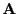matrix
• ACondin: the interval evaluation of the Condmatrix
• bin: the interval evaluation of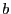• bCondin: the interval evaluation of Cond• bout: the enclosure of the interval linear system
• Param: the ranges for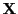• Func: a procedure that computes the interval evaluation of the elements of. These elements are stored rows by rows in an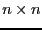interval vector (see the note 2.3.4.3)
• Jfunc: a procedure that computes the interval evaluation of the derivatives of the elements of, see the note 2.4.2.2
• bFunc:a procedure that computes the interval evaluation of the elements of, see the note 2.3.4.3
• Jbfunc: a procedure that computes the interval evaluation of the derivatives of the elements of, see the note 2.4.2.2

This procedure will return 1 if the Gauss elimination scheme has been completed, 0 otherwise. It will return in general a much more better enclosure than the classical interval Gauss elimination scheme.

For example consider the system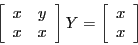for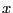in [3,4] andin [1,2]. Using the classical Gauss elimination scheme the enclosure of the solution is: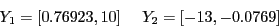while if we use the derivatives we get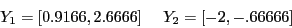while the solutions are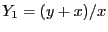(which has an enclosure of [1.25,1.666]),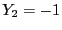.

The ALIAS-Maple procedure LinearBound implements this calculation.Next: Regularity of parametric interval Up: Solving systems of linear Previous: Mathematical background   Contents
Jean-Pierre Merlet 2012-12-20